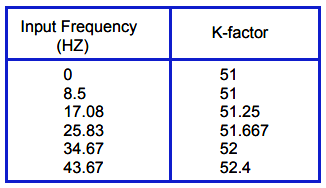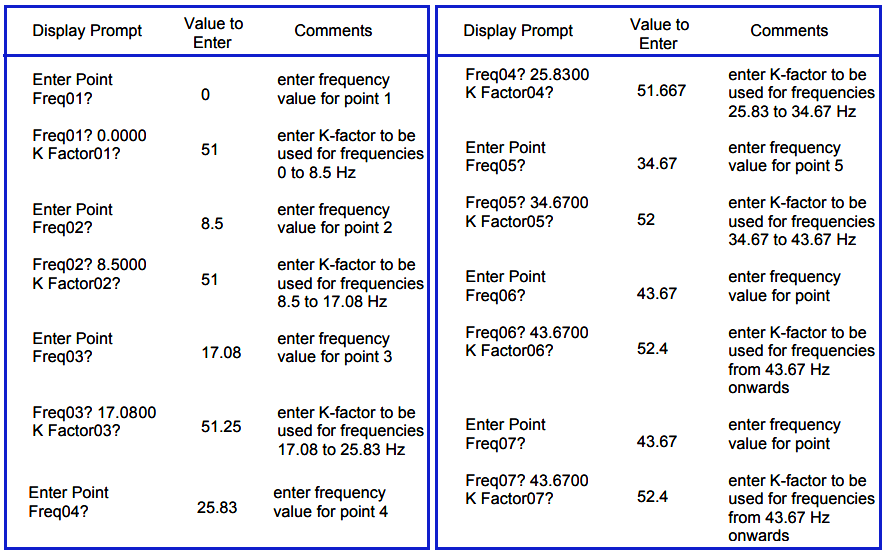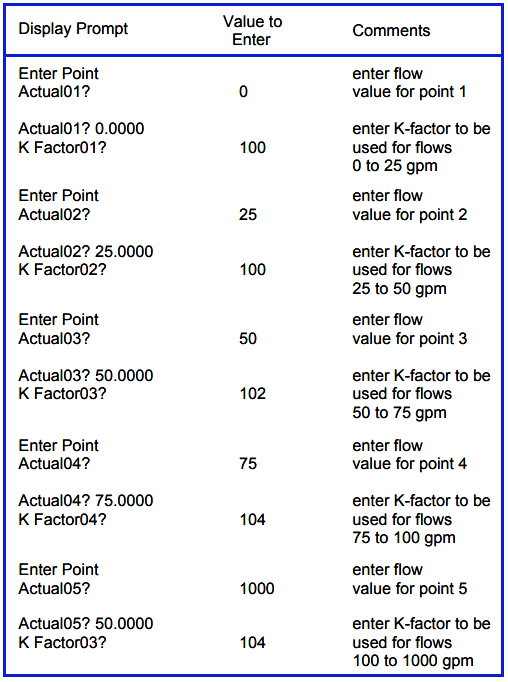# Flow Meter K-factor and Calculations

## Flow Meter K-factor :

The axial design of Turbine Flow Meter is inherently linear within a known turndown range, typically 15:1, based on velocity of the measured fluid.

The device has unmatched capability of precise and repeatable K-Factor generation based on the turning of the balanced rotor and the subsequently generated frequency pulse signal via the magnetic coil assembly, providing accuracy of +/-.15% with special calibration applied.

Each pulse generated represents a discrete amount of volumetric throughput. Dividing the total number of pulses generated by the specific amount of liquid product that passed through the PTF determines the K-Factor.

Put in the simplest of terms, K-Factor is no more complicated than understanding that the meter will generate a specific number of pulses for every unit of product passing through it. If the user can detect the pulses, then it is a straightforward task to determine flow rate and totals.

The K-Factor, expressed in pulses per unit volume, may be used to electronically provide an indication of volumetric throughput directly in engineering units.

Mass flow can be accomplished by the addition of pressure and temperature sensors that are factored electronically. The electronic device continuously divides the incoming pulses by the K-Factor, or multiplies them with the inverse of the K-Factor, to provide factored totalization, rate indication, and various outputs.

The frequency of the pulse output, or number of pulses per unit time, is directly proportional to the rotational rate of the turbine rotor.

Therefore, this frequency of the pulse output is proportional to the rate of the flow.

By dividing the pulse rate by the K-Factor, the volumetric throughput per unit time of the rate of flow can be determined.

Standard electronic devices are commonly used to provide instantaneous totalization and flow rate indication.

Plotting the electrical signal output versus flow rate provides the characteristics profile or calibration curves for the PTF.

Although the concept of K-Factor is applied widely with other types of meters, it should be noted that this value is calculated from analog values in other meters where a magnetic coil assembly reading a turning rotor is not used.

This calculation has built-in error factors based on a relatively imprecise primary input coupled with conversions from analog frequency and sometimes back to analog for transmission purposes.

Also See : Turbine Flow meter Animation

## Calculations Using K-Factors

### 1. What is a K-factor

Simply stated a K-factor is a dividing factor. The term is usually encountered when dealing with pulse signals although analog K-factors are sometimes used.

### 2. Pulse Signal K-factors

All pulse output type flow meters when they are dispatched by their manufacturer will have a calibration certificate.

The calibration certificate will show that the meter has been calibrated over its flow range and noted on the certificate will be the average K-factor for the meter.

This K-factor will be given in terms of the number of pulses produced by the meter for a given volumetric flow. (e.g.) 200 pulses per U.S. gallon, 150 pulses per liter etc.

This K-factor is the value that is entered into a batch meter or indicator/totalizer in order to give a readout in engineering units.

#### Example 1

If the display on a rate meter is required in U.S. gallons per second, and the K-factor of the flow meter is 210 pulses per U.S. gallon, then the K-factor entered into the rate meter would be 210.

If a totalizer associated with the same flow meter was to be set up so as to totalize in U.S. gallons the totalizer K-factor would be 210.

If the totalizer was to be set to totalize in tenths of a gallon the K-factor would be 210/10 = 21

#### Example 2

If the display on a rate meter is required in U.S. gallons per minute, and the K-factor of the flow meter is 210 pulses per U.S. gallon, then the K-factor entered into the rate meter would be: 210/60 = 3.5

#### 3. K-factors for Analog Input Signals

When batching, indication or totalization has to be carried out using an analog input signal a flow meter first converts the 4 to 20 mA signal into a 0 to 10000 Hz. signal.

The K-factor is then calculated by relating the engineering unit equivalent of 20 mA to the 10000 Hz. signal.

#### Example 3

A vortex meter outputs 20 mA when the flow is 2000 U.S. gallons per minute, we wish to display the rate in gallons per minute. The rate K-factor is = 10000/2000 = 5

The value of the totalizer K-factor will depend on whether the flow rate was given in units per second, minute or hour and whether it is desired to totalize in whole units, tenths, hundredths etc.

If the flow rate was given in units per second the totalizer K-factor (for whole units) is obtained by multiplying the rate K-factor by 1.

If the flow rate was given in units per minute the totalizer K-factor (for whole units) is obtained by multiplying the rate K-factor by 60.

If the flow rate was given in units per hour the totalizer K-factor (for whole units) is obtained by multiplying the rate K-factor by 3600.

The totalizer K-factor in example 3 will be = 5 x 60 = 300 in order to totalize in gallons.

If we wished to totalize in tenths of a gallon the K-factor would be 5 x 60/10 = 30

#### Example 4

An electromagnetic flow meter outputs 20 mA when the flow is 20 liters per second, we wish to display the flow rate in liters per second and totalize in M³.

The rate K-factor is 10000/20 = 500

The totalizer K-factor will be 500 x 1/0.001 = 500000

#### 4. Multi-Point K-factors

Some flow applications dictate that multiple K-factors are used. Two applications that require multiple K-factors are:

• flow meters with non linear outputs
• wide turndown flow applications

Some Flow meters have an option available that allows the user to input from 3 to 16 K-factors.

This multi-point K-factor option is available for both pulse and analog inputs.

#### 5. Multi-Point K-factors for Pulse inputs

The first step is to calculate K-factors to cover each flow range. This is done by taking the information on the flow meter manufacturer’s calibration sheet and calculating the K-factors.

An alternative to using the manufacturers’ data is to conduct tests on site against a calibrated standard.

The second step is to relate an incoming frequency range from the flow meter to a given calculated K-factor.

The final step is to program these values into the instrument.

#### Example 5

A turbine flow meter has the following calibration data.From the above calibration sheet data we can relate the incoming frequency to the K-factor as follows.The 16 point K-factor would be programmed as follows.Note that because the K-factor for the last two points are the same, any frequency higher than 43.67 Hz will be modified by a K-factor of 52.4 The set up is now complete.

#### 6. Multi Point K-factors for Analog Inputs

The procedure for analog inputs is essentially the same as for pulse inputs.

The first step is to calculate K-factors to cover each flow range. This is done by taking the information on the flow meter manufacturer’s calibration sheet and calculating the K-factors.

An alternative to using the manufacturer’s data is to conduct tests on site against a calibrated standard.

The second step is to relate an incoming flow value from the flow meter to a given calculated K-factor.

The final step is to program these values into the instrument.

#### Example 6

A vortex flow meter has the following calibration data.

Base K-factor 10000/100 = 100Using the MASStrol as an example the K-factors would be programmed as follows.Note that the point after the final one should have a flow value entered that is very much higher than the true maximum flow rate of the meter.

Note also that as the last two K-factors are the same any flow above 100 gpm will be modified by a K-factor of 104. The setup is now complete.

Credits : Sponsler & Kessler-Ellis (KEP)

#### Articles You May Like :

Flow Meter Accuracy

Venturi Flow Meter Animation

Flow rate using Level Sensors

What is Restriction Orifice ?

Compare Orifice & Restriction Orifice

Don't Miss Our Updates
Be the first to get exclusive content straight to your email.
We promise not to spam you. You can unsubscribe at any time.

### 2 thoughts on “Flow Meter K-factor and Calculations”

1.Dear Manager
I need your help.

We have on the same GAS LINE 2 flowmeters for Gas Turbine.

The first near the Gas turbine is Corriolis Micro motion (as reference) and is measuring the flow of gas on kg/h.

The Second is little bit far to the gas Turbine and is an Fluidwell E018-P associated with turbinemeter BARTON 7403.

The K-factor for the turbinemeter is (on calibration certificate) : 25.52 pul/Gal (UK).
linearity : 0.30 %

I need to introduce the K-factor on the Fluidwell E018-P

Wich value I have to us……. 25.52 pulse/Gal or ……?

I need the same unit on the totalizer fluidwell for flowrate : kg/h
but for totalizer : I need to use : cubic meter

2.